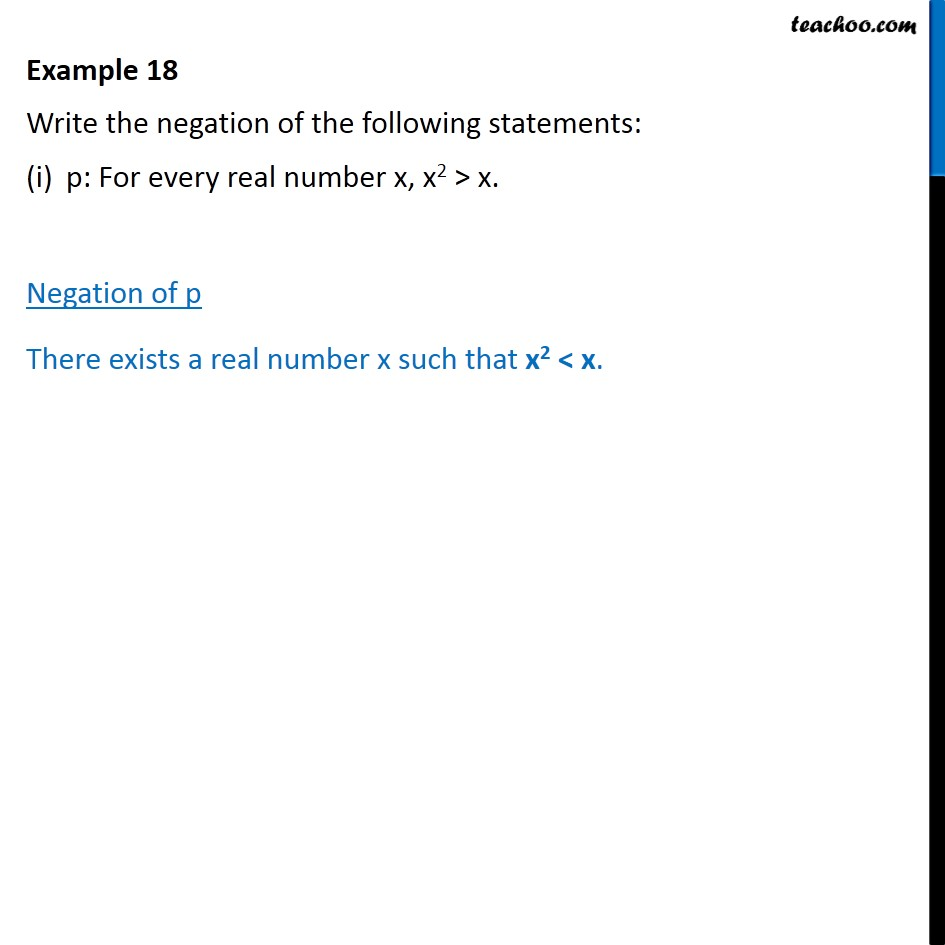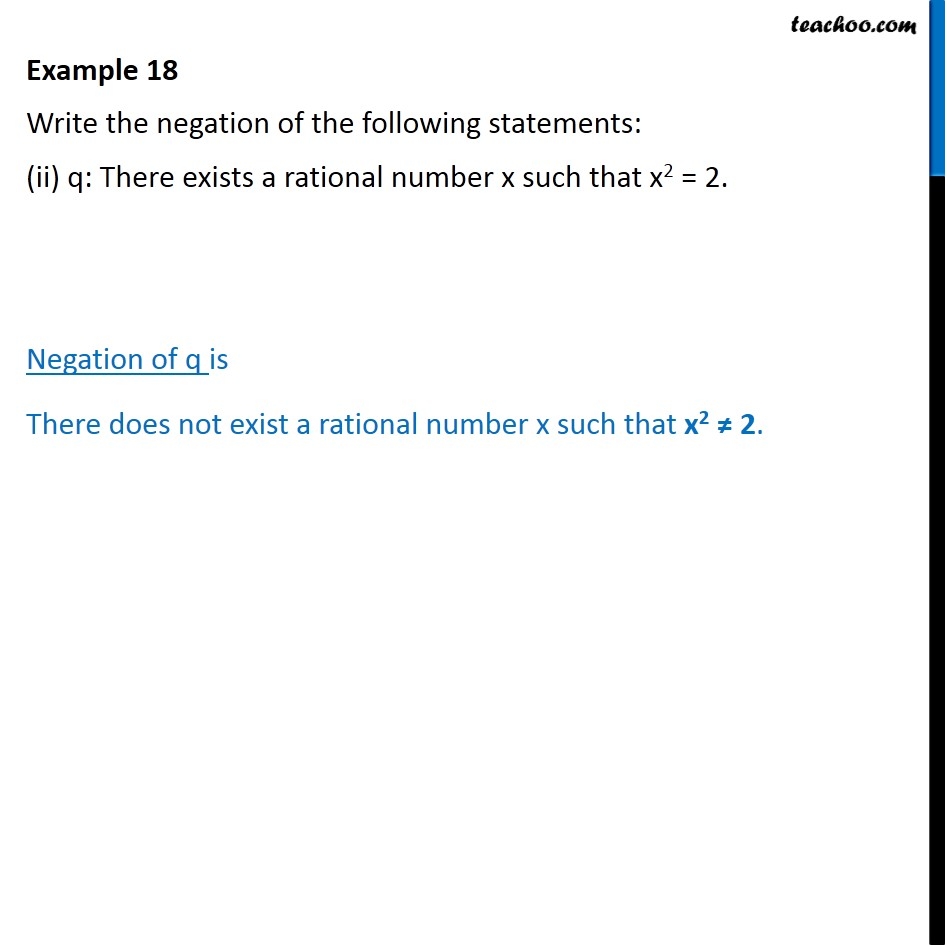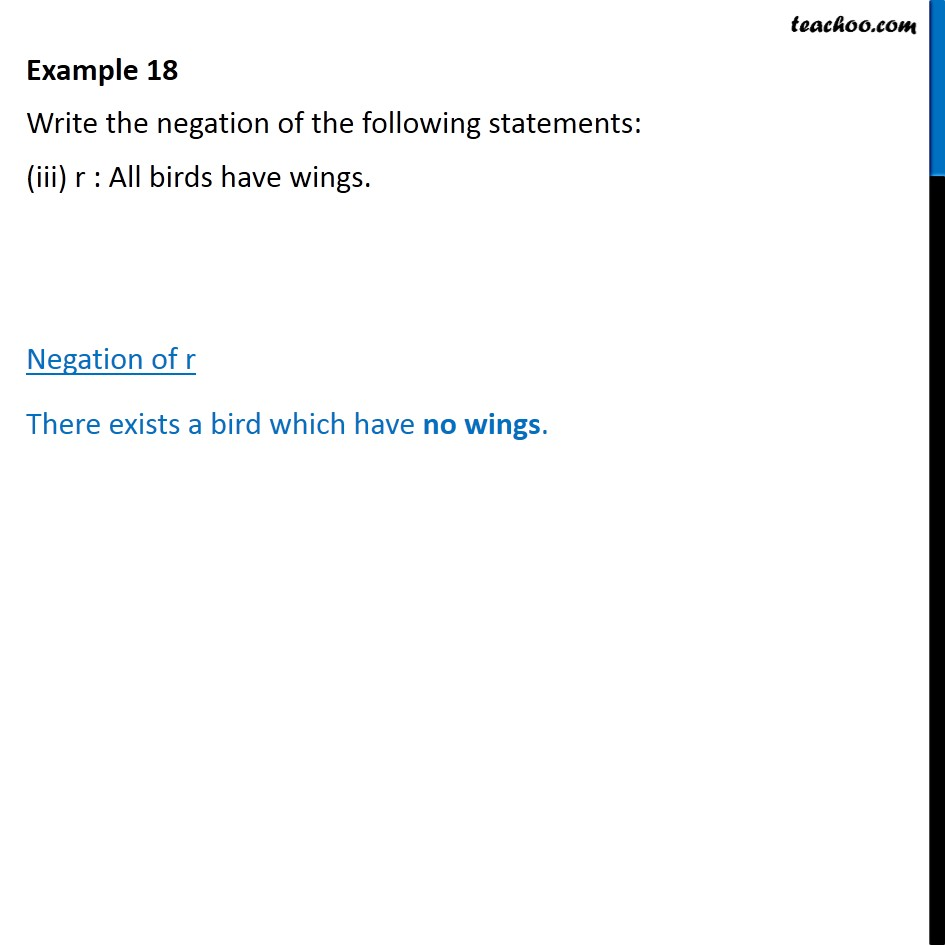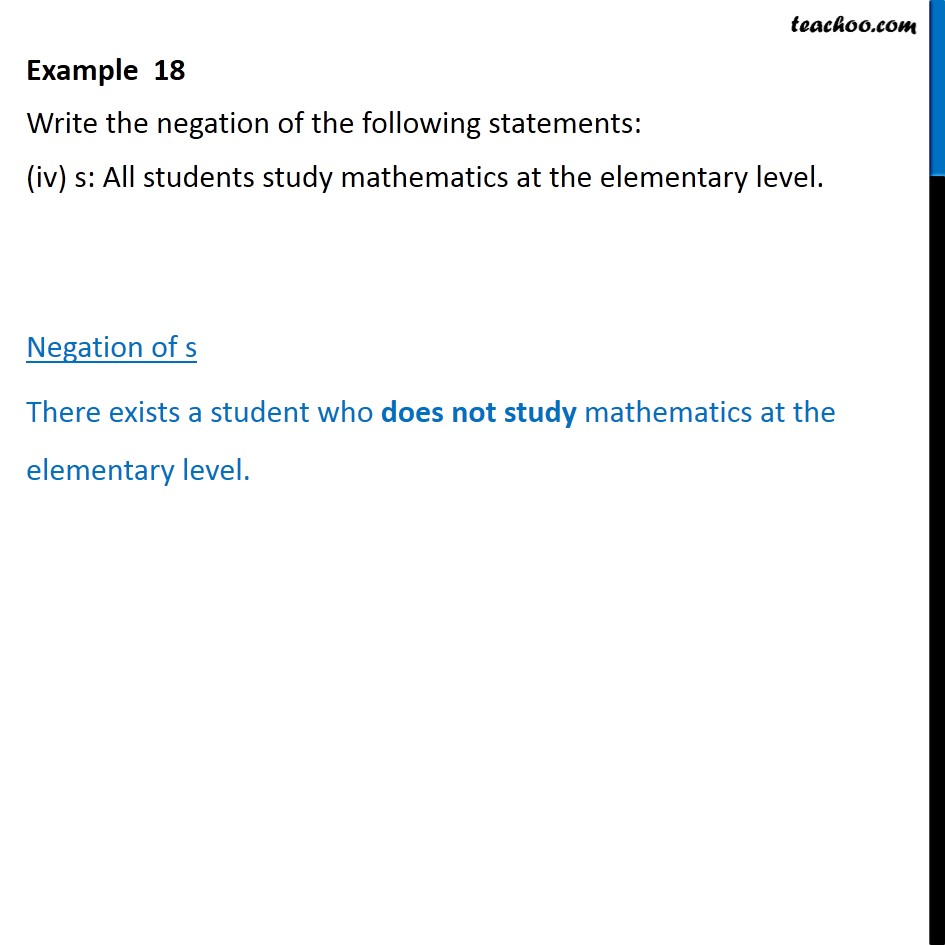1. Chapter 14 Class 11 Mathematical Reasoning (Deleted)
2. Serial order wise
3. Examples

Transcript

Example 18 Write the negation of the following statements: p: For every real number x, x2 > x. Negation of p There exists a real number x such that x2 < x. Example 18 Write the negation of the following statements: (ii) q: There exists a rational number x such that x2 = 2. Negation of q is There does not exist a rational number x such that x2 2. Example 18 Write the negation of the following statements: (iii) r : All birds have wings. Negation of r There exists a bird which have no wings. Example 18 Write the negation of the following statements: (iv) s: All students study mathematics at the elementary level. Negation of s There exists a student who does not study mathematics at the elementary level.

Examples